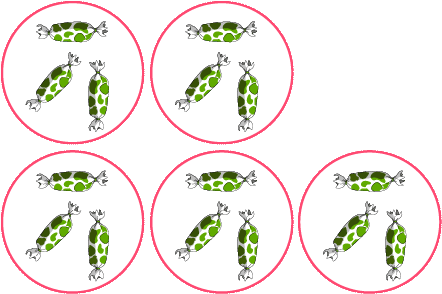# Equal Groups Multiplication | Understand multiplication Concept

## Multiplication equal groups test and printable worksheets

Equal groups multiplication is the best and basic way to solve multiplication problems with no stress. This is because the equal groups are usually presented with visually stimulating models, which can enable kids to understand multiplication concept in a sweet and easy way.

## Click the sections below to find more explanation on this topic

...

Mastering the multiplication concepts is a perfect way for kids to boast their strength in math.

The test on multiplication equal groups will in another way connect your kids to several familiar concepts, such as repeated addition.

Multiplication means you have a certain number of groups of equal size. The certain number of groups can be said to be 5, while the same group size may be 3s.
Thus 5 x 3, which also means 5 groups of 3, hence 3 + 3 + 3 + 3 + 3.### Objective - Multiplication equal group test and printable worksheets

The main objective in our multiplication equal groups test and printable worksheets below, is for kids are to count equal groups and fill in the blank spaces.

These blank spaces to be filled contain the number of groups of apples and the number of apple in each group.

Equally important, our aim of designing such a beautiful idea is to know how well kids can interpret various descriptions of equal group, and to evaluate their understanding of multiplication.

As a matter of fact, these designs are going to enable kids practice counting in a more special and effective way.

### Example - easy way to obtain number of groups and group size

Now we have as example 3 groups of apples and 4 apples in each group.This example above has simply produced our multiplication sentence of 3 x 4

How can we then obtain the total number of apples?

It is very easy. Just think of repeated addition, or three groups of 4s. It will however be. 4 + 4 + 4 = 12.

Wow! I really like this. It is very simple. 3 x 4 = 12

In another way, we could decide to count the number of apples one by one, beginning from the first group.
Here we go; 1, 2, 3, 4, 5, 6, 7, 8, 9, 10, 11, 12.